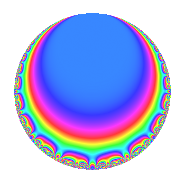# Properties

 Label 36.2.eLevel 36 Weight 2 Character orbit e Rep. character $$\chi_{36}(13,\cdot)$$ Character field $$\Q(\zeta_{3})$$ Dimension 2 Newforms 1 Sturm bound 12 Trace bound 0

# Related objects

## Defining parameters

 Level: $$N$$ = $$36 = 2^{2} \cdot 3^{2}$$ Weight: $$k$$ = $$2$$ Character orbit: $$[\chi]$$ = 36.e (of order $$3$$ and degree $$2$$) Character conductor: $$\operatorname{cond}(\chi)$$ = $$9$$ Character field: $$\Q(\zeta_{3})$$ Newforms: $$1$$ Sturm bound: $$12$$ Trace bound: $$0$$

## Dimensions

The following table gives the dimensions of various subspaces of $$M_{2}(36, [\chi])$$.

Total New Old
Modular forms 18 2 16
Cusp forms 6 2 4
Eisenstein series 12 0 12

## Trace form

 $$2q - 3q^{5} + q^{7} - 6q^{9} + O(q^{10})$$ $$2q - 3q^{5} + q^{7} - 6q^{9} - 3q^{11} + q^{13} + 9q^{15} + 12q^{17} - 8q^{19} + 3q^{21} + 3q^{23} - 4q^{25} - 3q^{29} - 5q^{31} - 9q^{33} - 6q^{35} + 4q^{37} - 3q^{39} - 3q^{41} + q^{43} + 9q^{45} + 9q^{47} + 6q^{49} - 12q^{53} + 18q^{55} + 3q^{59} + 13q^{61} - 3q^{63} + 3q^{65} + 7q^{67} - 9q^{69} - 24q^{71} - 20q^{73} - 12q^{75} + 3q^{77} - 11q^{79} + 18q^{81} + 9q^{83} - 18q^{85} - 9q^{87} + 12q^{89} + 2q^{91} + 15q^{93} + 12q^{95} - 11q^{97} + 9q^{99} + O(q^{100})$$

## Decomposition of $$S_{2}^{\mathrm{new}}(36, [\chi])$$ into irreducible Hecke orbits

Label Dim. $$A$$ Field CM Traces $q$-expansion
$$a_2$$ $$a_3$$ $$a_5$$ $$a_7$$
36.2.e.a $$2$$ $$0.287$$ $$\Q(\sqrt{-3})$$ None $$0$$ $$0$$ $$-3$$ $$1$$ $$q+(1-2\zeta_{6})q^{3}+(-3+3\zeta_{6})q^{5}+\zeta_{6}q^{7}+\cdots$$

## Decomposition of $$S_{2}^{\mathrm{old}}(36, [\chi])$$ into lower level spaces

$$S_{2}^{\mathrm{old}}(36, [\chi]) \cong$$ $$S_{2}^{\mathrm{new}}(18, [\chi])$$$$^{\oplus 2}$$